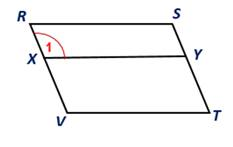Chapter 4.1, Problem 29EElementary Geometry For College St...

7th Edition
Alexander + 2 others
ISBN: 9781337614085

Solutions

Chapter
SectionElementary Geometry For College St...

7th Edition
Alexander + 2 others
ISBN: 9781337614085
Textbook Problem

In Exercises 27 to 30, use the definition of a parallelogram to complete each proof.Given: Parallelogram RSTV; also X Y - ∥ V T - Prove: ∠ 1 ≅   ∠ S Plan: First show that RSYX is a parallelogram.To determine

To prove:

1 S in the given figure.

Explanation

Given:

Parallelogram RSTV; also XY-VT-.

Corollary:

1. A parallelogram is a quadrilateral in which both pairs of opposite sides are parallel.

2. Opposite angles of a parallelogram are congruent.

Approach:

From the given parallelogram RSTV, and XY-VT-.

Here RS-VT- and XY-VT

Still sussing out bartleby?

Check out a sample textbook solution.

See a sample solution

The Solution to Your Study Problems

Bartleby provides explanations to thousands of textbook problems written by our experts, many with advanced degrees!

Get Started

Solve the equations in Exercises 126. 2x3x2x3x+1=0

Finite Mathematics and Applied Calculus (MindTap Course List)

Finding Derivatives In Exercises 51-62, find f(x). f(x)=2x23x+1x

Calculus: An Applied Approach (MindTap Course List)

29. Find what value of c is the function a probability density function.

Mathematical Applications for the Management, Life, and Social Sciences